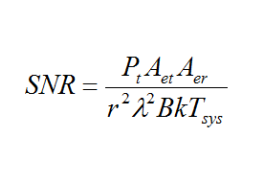#### Signal to noise ratio toolThe equation that is used when calculating SNR.

The signal-to-noise-ratio (SNR) is a criterion of detectability in a communication link. In basic terms, it compares the level of the desired signal to the level of background noise. A ratio in excess of 1:1 indicates more signal than noise.

The equation used in this tool is show in the image above. The parameters used in the equation are transmitted power (Pt), transmitter antenna effective aperture (Aet), receiver antenna effective aperture (Aer), transmitter-receiver distance (r), wavelength (λ), bandwidth (B), Boltzmann constant (k) and system temperature.

For example, the following inputs parameters will result in a signal to noise ratio (SNR) of 72.78e-3.

Transmit power: 1 mW

Operating requency: 1 GHz

Effective trasmitter antenna apaerture (AET): 78.53 cm2

Effective receiver antenna apaerture (AET): 100 cm2

Antenna separation: 10 Km

System bandwidth: 30 MHz

System temperature: 290 K